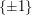## Пятница 25.10. Дмитрий Соколов: "(Semi)Algebraic proofs over $\{\pm 1\}$ variables"

Пятница, 25 октября, ауд. 106. Начало в 17:00.

Докладчик: Дмитрий Соколов.

Тема: (Semi)Algebraic proofs overvariables.

### Abstract

In this talk, we study versions of Polynomial Calculus and Sum-of-Squares proof systems. Current techniques for size lower on these proof systems bounds significantly use {0, 1} encoding of variables. More formally, we need to be able to assign any variable by a feasible value in a way to kill a term, that helps us to reduce a question about size to a question about degree.

We know the for Fourier''encoding of boolean variables degree lower bound is not enough to prove size lower bounds [Buss et al. 2001]. In this talk we present show exponential lower bounds on monomial size of proofs in SoS and PCR as well as separation between these systems. It is a solution to an open problem from [Impagliazzo, Mouli, Pitassi 2019].